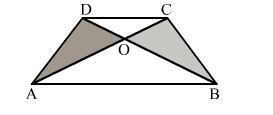# In the adjoining figure, ABCD is a trapezium in which AB || DC and its diagonals AC and BD intersect at O.

Question:

In the adjoining figure, ABCD is a trapezium in which AB || DC and its diagonals AC and BD intersect at O. Prove that ar(AOD) = ar(BOC).Solution:

CDA and ​∆CBD lies on the same base and between the same parallel lines.
So, ar(​∆CDA) = ar(CDB)            ...(i)
Subtracting ar(​∆OCD) from both sides of equation (i), we get:
ar(​∆CDA-">- ar(​∆OCD) = ar(​​∆CDB-">- ar (​​∆OCD)
⇒ ar(​​∆AOD) = ar(​​∆BOC)